# HSSlive: Plus One & Plus Two Notes & Solutions for Kerala State Board

## इयत्ता दहावी विज्ञान धडा रासायनिक अभिक्रिया आणि समीकरणे मराठी स्वाध्याय PDFइयत्ता दहावी विज्ञान धडा रासायनिक अभिक्रिया आणि समीकरणे मराठी स्वाध्याय PDF

या लेखात, आम्ही विज्ञान धडा रासायनिक अभिक्रिया आणि समीकरणे विषयासाठी इयत्ता दहावी मराठी सोल्यूशन्स देऊ. इयत्ता दहावी मधील विद्यार्थी पाठ्यपुस्तकांमध्ये उपस्थित असलेल्या व्यायामांसाठी प्रश्न आणि उत्तरे डाउनलोड आणि कॉपी करण्यास सक्षम असतील.

इयत्ता दहावी विज्ञान धडा रासायनिक अभिक्रिया आणि समीकरणेाच्या पुस्तकात महाराष्ट्र बोर्डाच्या अभ्यासक्रमातील सर्व प्रश्नांचा समावेश आहे. येथे सर्व प्रश्न पूर्ण स्पष्टीकरणासह सोडवले आहेत आणि डाउनलोड करण्यासाठी विनामूल्य उपलब्ध आहेत. महाराष्ट्र बोर्ड इयत्ता दहावी विज्ञान धडा रासायनिक अभिक्रिया आणि समीकरणेाचे पुस्तक खाली दिले आहे. आम्‍हाला आशा आहे की आमच्‍या इयत्‍ता दहावी वीच्‍या विज्ञान धडा रासायनिक अभिक्रिया आणि समीकरणेाचे पुस्‍तक तुमच्‍या अभ्यासात मदत करेल! जर तुम्हाला आमचे इयत्ता दहावी चे पुस्तक आवडले असेल तर कृपया ही पोस्ट शेअर करा.

## इयत्ता दहावी विज्ञान धडा रासायनिक अभिक्रिया आणि समीकरणे स्वाध्याय

 मंडळाचे नाव Maharashtra Board ग्रेडचे नाव दहावी विषय विज्ञान धडा रासायनिक अभिक्रिया आणि समीकरणे वर्ष 2022 स्वरूप PDF/DOC प्रदाता hsslive.co.in अधिकृत संकेतस्थळ mahahsscboard.in

## समाधानासह महाराष्ट्र बोर्ड आठवा स्वाध्याय कसे डाउनलोड करायचे?

महाराष्ट्र बोर्ड दहावी स्वाध्याय PDF डाउनलोड करण्यासाठी खालील स्टेप्स फॉलो करा:

1. वेबसाइट- Hsslive ला भेट द्या. 'स्वाध्याय' लिंकवर क्लिक करा.
2. महा बोर्ड दहावी स्वाध्याय PDF पहा.
3. आता महाराष्ट्र बोर्ड दहावी स्वाध्याय तपासा.
4. डाउनलोड करा आणि भविष्यातील संदर्भांसाठी जतन करा.

## इयत्ता दहावी विज्ञान धडा रासायनिक अभिक्रिया आणि समीकरणे स्वाध्याय उपाय

इयत्ता दहावी स्वाध्याय मधील विद्यार्थी खालील लिंक्सवरून विज्ञान धडा रासायनिक अभिक्रिया आणि समीकरणेाचे उपाय डाउनलोड करू शकतील.

Question 1.
Choose the correct option from the bracket and explain the statement giving reasons :
(Oxidation, displacement, electrolysis, reduction, zinc, copper, double diplacement, decomposition)

a. To prevent rusting, a laver of ……… metal is applied on iron sheets.
To prevent rusting, a layer of zinc metal is applied on iron sheets.
The rusting of iron is an oxidation process. Due to corrosion of an iron a deposit of reddish substance (Fe2O3.H2O) is formed on it. This substance is called rust. To prevent corrosion, a layer of zinc metal (galvanisation) is applied on iron sheets.

b. The conversion or ferrous sulphate to ferric sulphate is …….. reaction.
The conversion of ferrous sulphate to ferric sulphate is an oxidation reaction.
When ferric ion is formed. from ferrous ion, the positive charge is increased by one unit. while this happens the rerrous ion loses one electron. A process in which a metal or its ion loses one or more electrons is called an oxidation.
2FeSO4 → Fe2(SO4)3
Fe2 + SO42- → 2Fe3+ + SO42-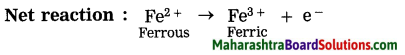c. When electric current is passed through acidulated water …….. of water takes place.
when electric current is passed through acidulated water decomposition of water takes place. In this reaction. hydrogen and oxygen gas are formed.

This decomposition takes place with the help of an electric current, it is also called electrolytic decomposition.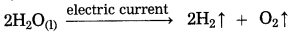d. Addition of an aqueous solution of ZnSO4 to an aqueous solution of BaCl2 is an example of ……… reaction.
Addition of an aqueous solution of ZnSO4 to an aqueous solution or BaCl2 is an example or double displacement reaction.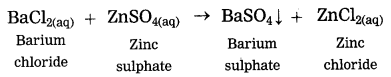Barium chloride reacts with zinc sulphate to form a white precipitate of barium sulphate. white precipitate is formed by exchange of ions Ba++ and SO4 between the reactants.

Question 2.
a. What is the reaction called when oxidation and reduction take place simultaneously? Explain with one example.
The reaction which involves simultaneous oxidation and reduction is called an oxidation-reduction or redox reaction.
In a redox reaction, one reactant gets oxidised while the other gets reduced during a reaction.
Redox reaction = Reduction + Oxidation

In redox reaction, the reductant is oxidized by the oxidant and the oxidant is reduced by the
reductant.
Example:CuO(s) + H2(g) → Cu(s) + H2O
In this reaction, oxygen is removed from copper oxide therefore it is a reduction of CuO, while hydrogen accepts oxygen to form water that means oxidation of hydrogen takes place. Thus oxidation and reduction reactions occur simultaneously.

Other examples of redox reactions: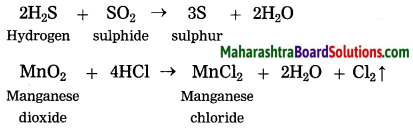b. How can the rate of the chemical reaction, namely, decomposition of hydrogen peroxide be increased?
At room temperature, the decomposition of hydrogen peroxide into water and oxygen takes place slowly. However, the same reaction occurs at a faster rate on adding manganese dioxide (MnO2),
powder in it.

c. Explain the term reactant product giving examples.

1. The substance which undergoes bond breaking while taking part in a chemical reaction is called reactant.
2. The substance formed as a result of a chemical reaction by formation of new bonds is called product.
3. Example: In a chemical reaction, the formation of carbon dioxide gas takes place by combustion of coal in air. In this reaction, coal (carbon) and oxygen (from air) are the reactants while carbon dioxide is the product.

d. Explain the types of reactions with reference to oxygen and hydrogen. Illustrate with examples.
With reference to oxygen and hydrogen, there are two types of reaction

1. Oxidation reaction
2. Reduction reaction.

1. Oxidation reaction:
Examples:
(1) When carbon burns in air, it forms carbon dioxide. In this reaction carbon accepts oxygen, therefore, this is an oxidation reaction.
C(s) + O2(g) → CO2(g)

(2) When sodium reacts with ethyl alcohol, sodium ethoxide and hydrogen gas is formed. In this reaction, hydrogen is removed from ethyl alcohol, therefore this is an oxidation reaction.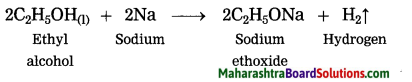(3) Acidified potassium dichromate (K2Cr2O7 / H2SO4) oxidises ethly alcohol to acetic acid.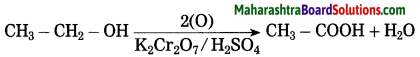2. Reduction reaction:
Examples:
(1) When hydrogen gas is passed over black copper oxide a reddish coloured layer of copper is
formed.
In this reaction an oxygen atom removed from CuO to form copper, hence, this is reduction.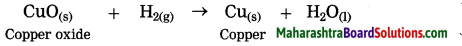(2) when hydrogen gas is passed over red hot coke, methane is obtained.
Here, hydrogen is added to coke (carbon). Hence, this is reduction.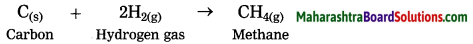e. Explain the similarity and difference in two events, namely adding NaOH to water and adding CaO to water.
Similarity : Both NaOH and CaO, when dissolved separately in water, solid NaOH dissolves releasing heat, resulting in rise in temperature. This reaction is exothermic reaction. When solid CaO dissolves in water, Ca(OH)2 is formed, large amount of heat is evolved. This reaction is also exothermic reaction. Both reactions are combination reactions and single product is obtained.
NaOH(s) + H2O → NaOH(aq) + Heat
CaO(s) + H2O → Ca(OH)2(aq) + Heat
Difference:

1. Aqueous solution of NaOH is considered as a strong alkali.
2. Aqueous solution of Ca(OH)2 is considered as a weak alkali.

Question 3.
Explain the following terms with examples.
a. Endothermic reaction
Endothermic reaction: The reaction in which heat is absorbed is called an endothermic
reaction.
when KNO3(s) dissolves in water, there is absorption of heat during the reaction and the temperature of the solution falls.
KNO2(s) + H2O(l) + Heat → KNO3(aq)

b. Combination reaction
When two or more reactants combine in a reaction to form a single product, it is called a combination reaction.
Examples:
1. The ammonia gas reacts with hydrogen chloride gas to form the salt in gaseous state, immediately it condenses at room temperature and gets transformed into the solid state.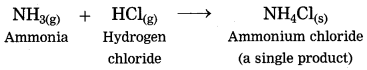2. Magnesium burns in air to form white powder of magnesium oxide as a single product.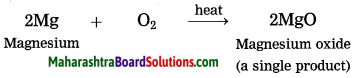3. Iron reacts with sulphur to form iron sulphide.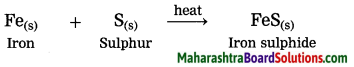c. Balanced equation
In a chemical reaction, the number of atoms of the elements in the reactants is same as the number or atoms of those elements in the product, such an equation is called a balanced equation.
Example: AgNO3 + NaCl → AgCl + NaNO3
In the above reaction, the number of atoms of the elements in the reactants is same as the number of atoms of elements in the products.

d. Displacement reaction
The reaction in which the place of the ion of a less reactive element in a compound is taken by another more reactive element by the formation of its own ions is called displacement reaction.

When zinc granules are added to the blue coloured copper sulphate solution, the zinc ions formed from zinc atoms take the place of Cu2+ ions in CuSO4, and copper atoms, formed from Cu2+ ions comes out i.e. the more reactive zinc displaces the less reactive Cu from copper sulphate.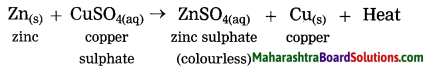4. Give scientific reason:
a. When the gas formed on heating lime stone is passed through freshly prepared lime water, the lime water turns milky.
when lime stone is heated, calcium oxide and carbon dioxide are formed. This carbon dioxide gas is passed through freshly prepared lime water, insoluble calcium carbonate and water are formed. In this reaction, lime water turns milky.b. It takes time for pieces of Shahabud tile to disappear in HCl, but its powder disappears rapidly.
The rate of a reaction depends upon the size of the particles of the reactants taking part in the reaction. The smaller the size of the reactants particles, the more is their total surface area and the faster is the rate of reaction.

In the reaction of dil. HCl with pieces of Shahabad tile, CO2 effervescence is formed amid the tile disappears slowly. On the other hand. CO2 effervescence forms at faster rate with Shahabad tile powder and it disappears rapidly.

c. While preparing dilute sulphuric acid from concentrated sulphuric acid in the laboratory, the concentrated sulphuric acid is added slowly to water with constant stirring.
(1) The preparation of dilute sulphuric acid falls in the category of extreme exothermic process.

(2) During the preparation of dilute sulphuric acid. large amount of water is taken in a glass container which is surrounded by ice. Cool it for twenty minutes, Now small quantity of conc. H2SO4 is added slowly with stirring. Therefore, only a small amount of heat is liberated at a time. In this way dilute sulphuric acid is prepared.

(3) On the other hand, in the process of dilution or conc. sulphuric acid with water, very large amount of heat is liberated. As a result, water gets evaported instantaneously, if it is poured in to conc. H2SO4 which may cause an accident.

d. It is recommended to use air tight container for storing oil for long time.

1. If edible oil is allowed to stand for a long time, it undergoes air oxidation, it becomes rancid and its smell and taste changes.
2. Rancidity in the rood stuff cooked in oil or ghee is prevented by using antioxidants. The process of oxidation reaction of food stuff can also be slowed down by storing it in air tight container.

Question 5.
Observe the following picture a write down the chemical reaction with explanation.The rusting of iron is an oxidation process. The rust on iron does not form by a simple reaction between oxygen and iron surface. The rust is formed by an electrochemical reaction. Fe oxidises to Fe2O3. H2O on one part of iron surface while oxygen gets reduced to H2O on another part or surface, Different regions on the surface of iron become anode and cathode.
(1) Fe is oxidised to Fe2+ in the anode region.
Fe(s) → Fe2+ (aq) + 2e
(2) O2 is reduced to form water in the cathode region.
O2(g) + 4H(aq) + 4e— → 2H2O(l)

When Fe2+ ions migrate from the anode region they react with water and futher get oxidised to form Fe3+ ions.
A reddish coloured hydrated oxide is formed from Fe3+ ions. It is called rust. It collects on the
surface.
2Fe3+ (aq) + 4H2O(l) → Fe2O3. H2O(s) + 6H+ (aq)
Because of various components in the atmosphere, oxidation of metals takes place, consequently resulting in their damage. This is called ‘corrosion’. Iron rusts and a reddish coloured layer is formed on it. This is corrosion of iron.

Question 6.
Identify from the following reactions the reactants that undergo oxidation and reduction.
a. Fe + S → FeS
Fe + S → FeS
In this reaction, Iron (Fe) undergoes oxidation
and sulphur. (S) undergoes reduction.

b. 2Ag2O → 4Ag + O2
2Ag2O → 4Ag + O2
In this reaction, reduction of Ag2O takes place.

c. 2Mg + O2 → 2MgO
2Mg + O2 → 2MgO
In this reaction, oxidation of Mg takes place.

d. NiO + H2 → Ni + H2O
NiO + H2 → Ni + H2O
In this reaction, reduction of NiO takes place and oxidation of H2 takes place.

Question 7.
Balance the following equation stepwise.
a. H2S2O7(l) + H2O(l) → H2SO4(l)
Step 1: Rewrite the given equation as it is
H2S2O7(l) + H2O(l) → H2SO4(l)
Step 2: write the number or atoms of each element in the unbalanced equation on both sides of equations.

 Element Number of atoms in reactant (left side) Number of atoms in products (right side) H 4 2 S 2 1 O 8 4

Step 3: To equalise the number of hydrogen atoms, sulphur atoms and oxygen atoms we use 2 as the coemficient or factor in the product.

 Element Number of atoms in reactant (left side) Number of atoms in products (right side) H 4 2 × 2 S 2 1 × 2 O 8 4 × 2 Total 14 14

Now the equation becomes H2S2O7 + H2O → 2H2SO4
Now, count the atoms of each element on both sides of the equation. The number of atoms on both sides are equal. Hence, the balanced equation is
H2S2O7 + H2O → 2H2SO4
Now indicate the physical states of the reactants and products.
H2S2O7(l) + H2O(l) → 2H2SO4(l)

b. SO2(g) + H2S(aq) → S(s) + H2O(l)
Step 1:
Rewrite the given equation as it is
SO2(g) + H2S(aq) → S(s) + H2O(l)

Step 2:
Write the number of atoms of each element in the unbalanced equation on both sides of equations.

 Element Number of atoms in reactants (left side) Number of atoms in products (right side) S 2 1 O 2 1 H 2 2

The number of hydrogen atoms on both sides of the equation is same, therefore, equalise the number of sulphur atoms and oxygen atoms.

Step 3: To balance the number of sulphur atoms:

 Number of atoms of sulphur In reactants In products S2O H2S (S) Initially 1 1 1 To balance 1 1 1 × 2

To equalise the number of sulphur atoms, we use 2 as the factor in the product, now the equation becomes
SO2 + H2S → 2S + H2O

Step 4:
To equalise the number of oxygen atoms in the unbalanced equation.

 Number of atoms of oxygen In reactants (SO2) In products H2O Initially 2 1 To balance 2 1 × 2

To equalise the number of sulphur atoms, we use 2 as the factor in the product i.e. H2O, now the unbalanced equation becomes
SO2 + H2S → 2S + 2H2O

Step 5:
To equalise the number of hydrogen atoms in unbalanced equation:

 Number of atoms of hydrogen In reactants (H2S) In products (H2O) Initially 2 4 To balance 2 × 2 4

To equalise the number of hydrogen atoms we use 2 as the factor in the reactant i.e, H2S, now the unbalanced equation become
SO2 + 2H2S → 2S + 2H2O
Now, count the atoms of each element on both sides of the equation, there are less number of sulphur atoms in the product. Now equalise the sulphur atoms, the balanced equation becomes,
SO2 + 2H2S → 3S + 2H2O
Now indicate the physical states of reactants and products.
SO2(g) + 2H2S(aq) → 3S(s) + 2H2O(l)

c. Ag(s) + HCl(l) → AgCl ↓ + H
Step 1:
Rewrite the given equation as it is
Ag(s) + HCl(l) → AgCl ↓ + H2 ↑

Step 2:
write the number of atoms or each element in the unbalanced equation on both sides of equations.

 Element Number of atoms in reactants (left side) Number of atoms in products (right side) Ag 1 1 H 1 2 Cl 1 1

The number of silver and chlorine atoms on both sides of the equation are same, therefore, equalise the number of hydrogen atoms.

Step 3:
To balance the number of hydrogen atoms.

 Number of atoms of hydrogen In reactants HCl In products H2 Initially 1 2 To balance 1 × 2 2

To equalise the number of hydrogen atoms, we use 2 as the factor in the product HCl, now the unbalanced equation become
Ag(s) + 2HCl → AgCl + H2

Step 4:
To balance the number of chlorine atoms:

 Number of atoms of chlorine In reactants (2HCl) In products (AgCl) Initially 2 1 To balance 2 2 ×1

To equalise the number of chlorine atoms, we use 2 as the factor in the product AgCl. now the unbalanced equation becomes
Ag + 2HCl → 2AgCl + H2
Now count the atoms of each element on both sides of the equation, there are less number of silver atoms in the reactant. Now equalise the silver atoms, the balanced equation becomes
2Ag + 2HCl → 2AgCl + H2
Now indicate the physical states of the reactunts and products
2Ag(s) + 2HCl(l) → 2AgCl ↓ + H2 ↑

d. H2SO4(aq) + NaOH(aq) → Na2SO4(aq) + H2O(l)
Step 1:
Rewrite the given equation as it is
H2SO4(aq) + NaOH(aq) → Na2SO4(aq) + H2O(l)

Step 2:
write the number of atoms of each element in the unbalanced equation on both sides of the equation.

 Element Number of atoms in reactants Number of atoms in products Na 1 2 S 1 1 O 5 5 H 3 2

The number of oxygen atoms involved in different compounds on both sides (reactants and products) are equal. Therefore, balance the number of atoms of the second element, sodium.

Step 3:
To balance the number of sodium atoms:

 Number of atoms of sodium In reactants In products To begin with 1 (in NaOH) 2 (in Na2SO4) To balance 1 × 2 2

To equalise the number of sodium atoms, we use 2 as the factor of NaOH in the reactants. Now, the partly balanced equation becomes as follows
H2SO4 + 2NaOH → Na2SO4 + H2O

Step 4:
Now, balance the number of hydrogen atoms:

 Number of atoms of hydrogen In reactants In products To begin with (in H2SO4)2 (in NaOH) 2 (in H2O) To balance 4 2 × 2

To equalise the number of hydrogen atoms, we use 2 as the factor or H2O in the products. The equation then becomes
H2SO4 + 2NaOH → Na2SO4 + H2O
Now, count the atoms of each element on both sides of the equation. The number of atoms on both sides are equal. Hence, the balanced equation is
H2SO4 + 2NaOH → Na2SO4 + 2H2O
Now indicate the physical states of the reactants and the products.
H2SO4(aq) + 2NaOH(aq) → Na2SO4(aq) + 2H2O(l)

Question 8.
Identify the endothermic and exothermic reaction.
a. HCl + NaOH → NaCl + H2O + heat
Exothermic reaction.

b. 2KClO3(s)Δ2KCl(s)+3O2
Exothermic reaction.

c. CaO + H2O → Ca(OH)2 + heat
Exothermic reaction.

d. CaCO3(s)ΔCaO(s)+CO2
Exothermic reaction.

Question 9.
Match the column in the following table:

 Reactants products Type of chemical reaction BaCl2(aq) + ZnSO4(aq) H2CO3(aq) Displacement 2 AgCl(s) FeSO4(aq) + Cu(s) Combination CuSO4(aq) + Fe(s) BaSO4↓ + ZnCl2(aq) Decomposition H2O(l) + CO2(g) 2Ag(s) + Cl2(g) Double displacement

 Reactants products Type of chemical reaction BaCl2(aq) + ZnSO4(aq) BaSO4↓ + ZnCl2(aq) Double displacement 2 AgCl(s) 2Ag(s) + Cl2(g) Decomposition CuSO4(aq) + Fe(s) FeSO4(aq) + Cu(s) Displacement H2O(l) + CO2(g) H2CO3(aq) Combination

Project:
1. Prepare aqueous solutions or various solid salts available in the laboratory. Observe what happens when aqueous solution of sodium hydroxide is added to these. Prepare a chart of double displacement reactions based on these observation.

2. Observe and note the physical and chemical changes experienced in various incidents in your day to day 1ife.

Can you recall? (Text Book Page No.16)

Question 1.
what are the types of molecules of elements and compounds?
Elements are divided into three classes i.e. metals, nonmetals and metalloids. When two or more elements combine chemically in a fixed proportion by weight, a compound is formed. The properties of a compound are altogether different from those of the constitutional elements.

Question 2.
what is meant by valency of element?
The number of electrons that an atom of an element gives away or takes up while forming an ionic bond, is called the valency or that element.

Question 3.
What is the requirement for writing molecular formulae of different compounds?
How are the molecular formulae of the compounds written?
while writing the molecular formulae of different compounds, the symbol of the radicals and their valence should be known.
The number of the ions is written as subscript on the right of the symbol or the ion. By cross multiplication of valenceies chemical formula is obtained.

Find out (Text Book Page No. 44)
Question1.
How are the blackened silver utensils and patinated (greenish) brass utensils cleaned?
The blackened silver utensils and patinated (greenish) brass utensils are cleaned using baking soda, vinegar and lemon mix.

Use your brain power! (Text Book Page No. 35)

Question 1.
write down the physical states of reactants and products in the reaction
SO2 + 2H2S → 3S + 2H2O
Reactants : SO2(g), 2H2S(g)
Products : 3S(s), 2H2O(l).

Question 2.
write down the physical states of reactants and products in the reaction
2Ag + 2HCl → 2AgCl + H2
Reactants: 2Ag(s), 2HCl(l)
Products: 2AgCl ↓, H

Question 3.
Identify the reactants and products of the following equation.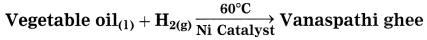Reactants: vegetable oil, H2(g)
Product: Vanaspathi ghee

Use your brain power! (Text Book Page No. 42)

Question 1.
Which is the oxidant used for purification of drinking water?
The chlorine based oxidants are used in the purification of drinking water.

Question 2.
Why is potassium permanganate used during cleaning water tanks?
Potassium permanganate is an oxidising agent. It oxidises dissolved iron, manganese and hydrogen sulphide into solid particles that are filtered out of the water tank. It is used to control iron bacteria growth in tank.

Can you tell? (Text Book Page No. 43)

Question 1.
what is the type of this reaction, in which Vanaspathi ghee is formed from vegetable oil?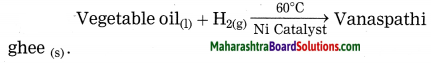In the preparation of vanaspathi ghee from vegetable oil hydrogen gas is used. This process is known as hydrogenation. This is reduction reaction.

Find out (Text Book page No. 33)

What are the other uses of silver nitrate in every day life?
Silver nitrate is used in the voters-ink. It is used as reactant in the laboratory. Silver nitrate is used to prevent infection in wounds and skin burns.

Use your brain power! (Text Book Page No. 35)

Question 1.
N2(g) + H2(g) ⇌ NH3(g)
Step 1:
Rewrite the given equation as it is
N2(g) + H2(g) ⇌ NH3(g)

Step 2:
Write the number of atoms of each element in the unbalanced equation on both sides of equations

 Element Number of atoms in reactants Number of atoms in products N 2 1 H 2 3

Step 3:
In the given equation. NH3 is a compound and it contains hydrogen element. On the left hand side there are two H atoms and on the right side 3H atoms. Equalise H atoms on both sides.

 Hydrogen atoms In reactants In products Initially 2 3 To balance 3 × 2 2 × 3

To equalise the number of hydrogen atoms, we use 3 as the factor in the reactant and 2 as the factor in the products. Now the equation becomes
N2 + 3H2 → 2NH3
Now, count the atoms of each element on both sides of the equation. The number of atoms on both sides are equal. Hence, the balanced equation is
N2 + 3H2 → 2NH3
Now indicate the physical states of the reactants and products
N2(g) + 3H2(g) ⇌ 2NH3(g)

Question 2.
Calcium chloride + Sulphuric acid → Calcium sulphate + Hydrogen chloride.
Step 1:
Write the chemical equation from the given word equation.
CaCl2 + H2SO4 → CaSO4 + HCl

Step 2:
Write the number of atoms of each element in the unbalanced on both sides of equation.

 Element Number of atoms in reactants Number of atoms in products Ca 1 1 Cl 2 1 H 2 1 S 1 1 O 4 4

Step 3:
In the given equation H2SO4 is a compound and it contains hygrogen element. On the left hand side there are two hydrogen atoms and on the right side one hydrogen atom. Equalise H atoms on both sides.

 Hydrogen atoms In reactants (H2SO4) In products (HCl) Initially 2 1 To balance 2 2  × 1

To equalise the number of hydrogen atoms we use 2 as the factor in the product so that the number of H atoms on both sides are equal. Therefore, the equation becomes
CaCl2 + H2SO4 → CaSO4 + 2 HCl
Now, count the atoms of each element on both sides of the equation. The number of atoms on both sides are equal hence, the balanced equation is
CaCl2 + H2SO4 → CaSO4 + 2 HCl
Now, indicate the physical state of the reactants and products.
CaCl2(s) + H2SO4(l) → CaSO4(s) + HCl(l)

Can you tell? (Text Book Page No. 39)

Take into account the time required for following processes. Classify them into two groups and give titles to the groups.
(1) Cooking gas starts burning on ignition.
(2) Iron article undergoes rusting.
(3) Erosion of rocks takes place to form soil.
(4) Alcohol is formed on mixing yeast in glucose solution under proper condition.
(5) Effervescence is formed on adding baking soda into a test tube containing dilute acid.
(6) A white precipitate is formed on adding dilute sulphuric acid to barium chloride solution.
The above processes are classified into two groups (a) slow speed reactions (b) fast speed reactions.
Slow speed reactions: (2), (3) and (4).
Fast speed reactions: (1),(5) and (6).

Use your brain power! (Text Book Page No. 43)

Question 1.
Some more examples of redox reaction are as follows. Identify the reductants and oxidants from them.
(1) 2H2S + SO2 → 3S↓ + 2H2O
(2) MnO2 + 4HCl → MnCl2 + 2H2O + Cl2
Oxidants: SO2, MnO2
Reductants: H2S, HCl

Question 2.
If oxidation means losing electrons, what is meant by reduction.
Reduction means gaining one or more electrons.

Question 3.
Write the reaction of formation of Fe2+ by reduction Fe3+ by making use of the symbol (e).
Fe3+ + e → Fe2+ (reduction)

Think about it (Text Book Page No. 43)

Question 1.
The luster of the surface of the aluminium utensils in the house is lost after a few days. Why does this happen?
The aluminium utensils when kept in the house for a few days, oxidation of aluminium takes place, a thin laver aluminium oxide (Al2O3) is deposited on the surface. Hence, aluminium utensils lose their lustre in a few days.

Question 3.
How many products are formed in each of the above reactions?
A single product is formed in each of the above reaction.

Use your brain power! (Text Book Page No. 39)

Question 1.
What is the difference in the process of dissolution and a chemical reaction.
In the process of dissolution, new substance is not necessarily formed. Whereas in a chemical reaction a new substance is definitely formed.

Question 2.
Does a new substance form when a solute dissolves in a solvent?
It is not necessary that a new substance is always formed.

Fill in the blanks:

Question 1.
Organic waste is decomposed by micro-organism and as a result manure and……..are formed.
Organic waste is decomposed by micro-organism and as a result manure and bio gas are formed.

Question 2.
……….is formed on mixing yeast in glucose solution under proper condition.
Alcohol is formed on mixing yeast in glucose solution under proper condition.

Question 3.
The chemical reaction during which H2(g) is lost is termed as………
The chemical reaction during which H2(g) s lost is termed as oxidation.

Question 4.
Corrosion can be prevented by using………
Corrosion can be prevented by using antirust solution.

Question 5.
The chemical reactions in which heat is liberated are called………..reactions.
The chemical reactions in which heat is liberated are called exothermic reactions.

Question 6.
The chemical formula of rust is………
The chemical formula of rust is Fe2O3.H2O.

Question 7.
A reaction in which heat is absorbed is called………reaction.
A reaction in which heat is absorbed is called endothermic reaction.

Question 8.
The process of rusting or iron is………process.
The process of rusting of iron is oxidation process.

Question 9.
when oil and fats are oxidised or even allowed to stand in air for a long time, they become ……….
when oil and fats are oxidised or even allowed to stand in air for a long time, they become rancid.

Question 10.
……… are used to prevent oxidation of food.
Antioxidants are used to prevent oxidation of food.

Question 11.
Carbon dioxide is passed through water. The reaction is a………reaction.
Carbon dioxide is passed through water. The reaction is a combination reaction.

Question 12.
Calcium carbonate is heated. The reaction is a………..reaction.
Calcium carbonate is heated. The reaction is a decomposition reaction.

Question 13.
Zinc strip is dipped in a CuSO4 solution. The reaction is a……….reaction.
Zinc strip is dipped in a CuSO4 solution. The reaction is a displacement reaction.

Question 14.
Silver nitrate solution is added to NaCl solution. The reaction is a……….reaction.
Silver nitrate solution is added to NaCl solution. The reaction is a double displacement reaction.

Question 15.
The slow process of decay or destruction of a metal due to effect of air, moisture and acids on it is known as……….
The slow process of decay or destruction of a metal due to effect of air, moisture and acids on it is known as corrosion.

Rewrite the following statements by selecting the correct options:

Question 1.
The reaction of iron nail with copper sulphate solution is………reaction. (March 2019)
(a) double displacement
(b) displacement
(c) combination
(d) decomposition
(b) displacement

Question 2.
Reddish brown deposit formed on iron nails kept in a solution of copper sulphate is
(a) Cu2O
(b) Cu
(c) CuO
(d) CuS
(b) Cu

Question 3.
The reaction CuSO4(aq) + Zn(s) → ZnSO4(aq) + Cu(s) is a……..reaction.
(a) displacement
(b) double displacement
(c) decomposition
(d) combination
(a) displacement

Question 4.
………is a combination reaction.
(a) Cu + H2SO4 → CuSO4 + H2
(b) H2 + Cl2 → 2HCl
(c) 2HgOΔ2Hg+O2
(d) CaCO3ΔCaO+CO2
(b) H2 + Cl2 → 2HCl

Question 5.
………..a decomposition reaction.
(a) CaCO3ΔCaO+CO2
(b) H2O + CO2 → H2CO3
(c) CaS + 2HCl → CaCl2 + H2S
(d) 2H2 + O2 → 2H2O
(a) CaCO3ΔCaO+CO2

Question 6.
In a chemical equation the……….are written on the left hand side.
(a) products
(b) reactants
(c) catalysts
(d) elements
(b) reactants

Question 7.
The Δ sign written above the arrow indicates………..of the reaction.
(a) reactant
(b) product
(c) heat
(d) direction of the reaction
(c) heat

Question 8.
The reaction KNO3(S) + H2O(l) + Heat → KNO3(aq) is a/an……….reaction.
(a) exothermic
(b) endothermic
(c) oxidation
(d) reduction
(b) endothermic

Question 9.
The reaction NaOH(S) + H2O(l) → NaOH(aq) is a/an……..reaction.
(a) exothermic
(b) endothermic
(c) oxidation
(d) reduction
(a) exothermic

Question 10.
A solution of Al2(SO4)3 in water is……….
(a) blue
(b) pink
(c) green
(d) colourless
(d) colourless

Question 11.
Carbon dioxide………..
(a) turns lime water milky
(b) is odourless
(c) is colourless
(d) All the three (a), (b) and (c) are correct
(d) All the three (a), (b) and (c) are correct

Question 12.
……….is the correct set up to pass CO2 through lime water.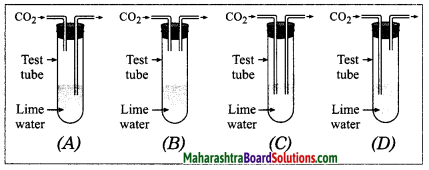Correct set up D.

Question 13.
when……..is passed through fresh lime water, it turns milky.
(a) H2
(b) CO
(c) CO2
(d) SO2
(c) CO2

Question 14.
Magnesium reacts with con. HCl to form………..salt.
(a) copper chloride
(b) ferrous chloride
(c) calcium chloride
(d) magnesium chloride
(d) magnesium chloride

Question 15.
Zinc reacts with hydrochloric acid. The reaction is a reaction.
(a) combination
(b) decomposition
(c) displacement
(d) double decomposition
(c) displacement

Question 16.
In a double displacement reaction,………… (Practice Activity Sheet – 1)
(a) ions remain at rest
(b) ions get liberated
(c) ions are exchanged
(d) ions are not created
(c) ions are exchanged

State whether the following statements are True or False:

Question 1.
Rusting of iron is a fast reaction.
False. (Rusting of iron is a slow reaction.)

Question 2.
Milk is set into curd is a chemical change.
True.

Question 3.
The reaction between salt and water is an example of exothermic reaction.
False. (The reaction between salt and water is an example of endothermic reaction.)

Question 4.
The speed of a chemical reaction depends on the catalyst used in the chemical reaction.
True.

Question 5.
The simple form of representation of a chemical reaction in words is known as word reaction.
True.

Question 6.
Nascent oxygen is always denoted by showing the symbol of oxygen.
False. (Nascent oxygen is always denoted by showing symbol of oxygen  in square brackets.)

Question 7.
Antioxidants are used to prevent oxidation or food containing fats and oils.
True.

Question 8.
When oils and fats are allowed to stand for a long time, they become rancid.
True.

Question 9.
The chemical formula of rust is Fe3O4 .xH2O.
False. (The chemical formula or rust is Fe2O.xH2O.)

Question 10.
Glucose combines with oxygen in our body and provides energy. The reaction is an endothermic reaction.
False. (Glucose combines with oxygen in our body and provides energy. The reaction is an exothermic reaction.)

Question 11.
Chemical reactions in which reactants gain oxygen are reduction reactions.
False. (Chemical reactions in which reactants gain oxygen are oxidation reactions.)

Question 12.
CuSO4(aq) + Znl(s) → ZnlSO4(aq) + Cu(s) is an example of decomposition reaction.
False. (It is an example of displacement reaction.)

Question 13.
The chemical reactions in which heat is liberated are called endothermic reactions.
False. (The chemical reactions in which heat is liberated are called exothermic reactions.)

Question 14.
The product or insoluble solid in chemical reaction is indicated by an arrow pointing upwards.
False. (The product or insoluble solid in chemical reaction is indicated by an arrow ↑ pointing downwards.)

Question 15.
The rate of a reaction increases on increasing the temperature.
True.

Question 16.
The digestion of food is a chemical decomposition process.
True.

Question 17.
The reaction between sodium hydroxide and hydrochloric acid is a slow reaction.
False (The reaction between sodium hydroxide and hydrochloric acid is a fast reaction.)

Question 18.
When calcium carbonate is heated, it decomposes into calcium oxide and oxygen gas.
False (when calcium carbonate is heated. it decomposes into calcium oxide and carbon dioxide gas.

Question 19.
The rate of a chemical reaction changes in presence of catalyst.
True.

Question 20.
Chlorines is an oxidant.
True.

Taking into consideration the relationship in the first pair, complete the second pair. (OR) Complete the following:

Question 1.
2H2 + O2 → 2H2O Combination reaction :: 2HgO → 2Hg + O2 :……….
Decomposition reaction

Question 2.
NH3 + HCl → NH4Cl : Combination reaction :: Fe + CuSO4 → FeSO4 + Cu :……..
Displacement reaction

Question 3.
2C2H5OH + 2Na → 2C2H5ONa + H2 : Oxidation :: CuO + H2 → Cu + H2O :……….
Reduction

Question 4.
CuCl2 + 2KI → CuI2 + 2KCl : Double displacement :: Zn + 2HCl → ZnCl2 + H2 :……….
Displacement reaction

Question 5.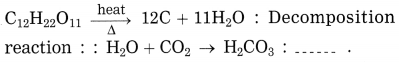Combination reaction

Question 6.
CuI2 : Brown :: AgCl :……….
White.

Match the column in the following table:

Question 1.

 Reactants products Type of chemical reaction Fe + S NaCl + H2O Oxidation CuSO4 + Zn 2CuO Neutralization 2Cu + O2 ZnSO4 + Cu Displacement HCl + NaOH FeS Combination

 Reactants products Type of chemical reaction Fe + S FeS Combination CuSO4 + Zn ZnSO4 + Cu Displacement 2Cu + O2 2CuO Oxidation HCl + NaOH NaCl + H2O Neutralization

Rewrite the second column so as to match the item from first column or Match the following:

Question 1.

 Column I Column II 1. Reduction (a) Type of a chemical reaction 2. Oxidation (b) Combination with hydrogen 3. Double displacement (c) Losing hydrogen 4. Displacement (d) Exchange of ions

(1) Reduction – Combination with hydrogen
(2) Oxidation – Losing hydrogen
(3) Double displacement – Exchange of ions
(4) Displacement – Type of chemical reaction.

Question 2.

 Column I Column II 1. Oils and fats are allowed to stand in air for a long time (a) Slow  reaction 2. NaOH dissolves in water (b) Rancid 3. Zinc is added to CuSO4 solution (c) Exothermic reaction 4. Rusting of water (d) Colourless Solution

(1) Oils and fats are allowed to stand in air for a long time – Rancid
(2) NaOH dissolves in water – Exothermic reaction
(3) Zinc is added to CuSO2 solution – Colourless solution
(4) Rusting of iron – Slow reaction.

Question 3.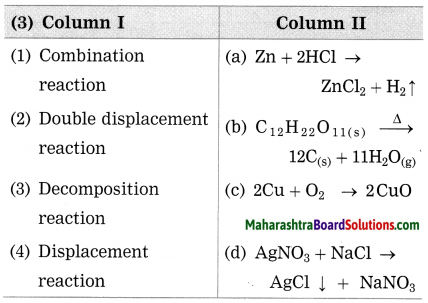(1) Combination reaction – 2Cu +O4 → 2CuO
(2) Double displacement reaction – AgNO3 + NaCl → AgCl ↓ + NaNO3
(3) Decomposition reaction – C12H22O11(s)Δ12C(s)+11H2O(g)
(4) Displacement reaction – Zn+ 2HCl → ZnCl2 + H

Classify each of the following reactions as combination, decomposition, displacement or double displacement reactions: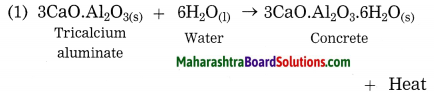Combination reaction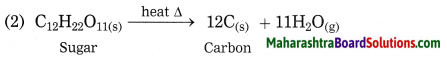Decomposition reaction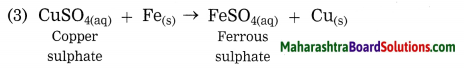Displacement reaction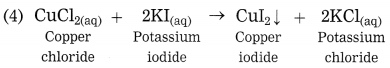double displacement reaction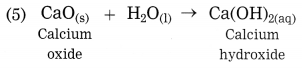Combination reaction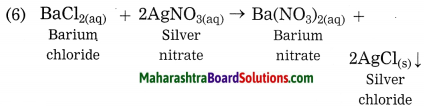double displacement reaction

Name the following:

Question 1.
The product formed in the thermal decomposition of sugar.
Carbon is formed in the thermal decomposition of sugar.

Question 2.
The gas evolved when sorghum metal reacts with ethanol.
Hydrogen (H2) gas is evolved when sodium metal reacts with ethanol.

Question 3.
The precipitate formed when barium sulphide reacts with zinc sulphate.
When barium sulphide reacts with zinc sulphide, a precipitate of barium sulphate is formed.
BaS+ZnSO4BaSO4 precipitate +ZnS

Question 4.
The reducing agent used for the reduction of copper oxide.
Hydrogen is used for the reduction of copper oxide.

Question 5.
The catalyst used to accelerate the rate of decomposition of hydrogen peroxide.
Manganese dioxide (MnO2) is used as a catalyst to accelerate the rate of decomposition of hydrogen peroxide.

Question 6.
which oxidising agent is used to oxidise ferrous sulphate.
Potassium permanganate (KMnO4) is used as an oxidising agent to oxidise ferrous sulphate.

Question 7.
The product formed in the oxidation of ethyl alcohol.
Acetic acid is formed in the oxidation of ethyl alcohol.

Answer the following questions in one sentence each:

Question 1.
what is meant by a chemical equation?
The simple representation or a chemical reaction in a condensed form with the help of chemical formulae is called a chemical equation.

Question 2.
what is meant by a word equation?
The simple form or representation or a chemical reaction in words is known as word equation.

Question 3.
what happens in a combination reaction?
A single compound (product) is formed from two or more substances during a combination reaction.

Question 4.
what happens in a displacement reaction?
In a displacement reaction. a more reactive element displaces another element, having less reactivity, from its compound.

Question 5.
what happens in a decomposition reaction?
A single substance is broken down and two or more substances are formed during a decomposition reaction.

Question 6.
what happens in a double displacement reaction?
A precipitate is formed by exchange of ions between the reactants during a double displacement reaction.

Question 7.
IdentIry the type of following reaction:
C12H22O11Δ12C+11H2O (Practice Activity Sheet – 2)
The above reaction is a decomposition reaction.

Question 8.
what happens in an endothermic reaction?
In an endothermic reaction, the reactants absorb heat to form products.

Question 9.
State the use of antioxidants in food containing fats and oils.
Antioxidants are used to prevent oxidation of food containing fats and oils.

Question 10.
What are edible oils?
Edible oils are compounds of alcohols and organic acids (carboxylic acids). The compounds formed are known as esters of carboxylic acids.

Question 11.
Is rancidity a phenomenon of oxidation or reduction?
Rancidity is a phenomenon of oxidation.

Question 1.
What do you understand by a physical change?
OR
Define physical change.
The change in which only the physical state of a substance is changed; no new substance is formed. This change is temporary. During this change the composition of the substance does not change.

Question 2.
Explain giving two examples or physical change.
(1) Conversion of ice into water is a physical change. On heating, ice melts into water. when the water is cooled, it freezes into ice. Thus, we get ice from water by a simple method and no new substance is formed. Hence, conversion of ice into water is a physical change.

(2) Magnetization of iron nail is a physical change. An iron nail magnetized by induction loses its magnetism as soon as it is detached from the magnet which induces magnetism in it. An iron nail magnetized by some other methods can also be demagnetized by simple means such as hammering or heating it. Thus, the magnetization of an iron nail can be easily reversed to get original nail. Hence, it is a physical change.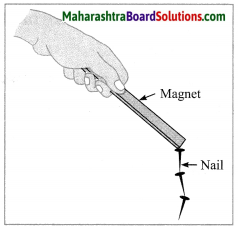Question 3.
what do you understand by a chemical change?
OR
Define Chemical change.
The change in which a substance or substances are converted into a new substance or substances, possessing properties altogether different from the original ones, is called a chemcial change. During this change, the original substance cannot be recovered by any simple means. This change is permanent.

Question 4.
Explain giving two examples of chemical change.
(1) When carbon is burnt, carbon dioxide is formed. In this process carbon combines with oxygen, therefore carbon and oxygen are reactants, while curbon dioxide is a product. This change is permanent.
C(s)+O2(g) Heat CO2(g)

(2) When a magnesium wire is burnt in air, a white powder of magnesium oxide is formed. We cannot obtain magnesium from magnesium oxide by simple methods. Properties of magnesium oxide are altogether different from those of magnesium. A new substance MgO is formed in the reaction. Hence, this change is a chemical change.
2Mg Magnesium +O2Δ2MgO Magnesium oxide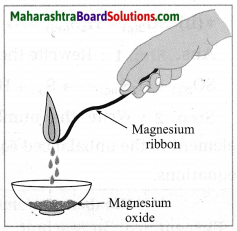Question 5.
What is meant by a chemical reaction?
A process in which some substances undergo bond breaking and are transformed into new substances by formation of new bonds is called a chemical reaction.

Question 6.
What is the importance of a chemical equation?

1. Reactants are converted into products.
2. Mass is conserved.
3. Atoms are conserved.
4. The properties and compositions of the products of a chemical reaction are different from those of its reactants.
5. Generally, energy is either absorbed or evolved.

Question 7.
What are the conventions used in writing a chemical equation?
Conventions used in writing a chemical equation:
(1) The reactants are written on the left hand side (LHS), while the products are written on the right hand side (RHS).

(2) Whenever there are two or more reactants, a plus sign (+) is written between each two of them. Similarly, if there are two or more products, a plus sign is written between each two of them.

(3) Reactant side and product side are connected with an arrow (→) pointing from reactants to products. The arrow represents the direction of the reaction. Heat is to be given from outside to the reaction, it is indicated by the sign Δ written above the arrow.

(4) The conditions like temperature, pressure, catalyst, etc., are mentioned above the arrow (→) pointing towards the product side.

(5) The physical states of the reactants and products are also mentioned in a chemical equation. The notations g, l, s, and aq are written in brackets as a subscript along with the symbols / formulae of reactants and products. The symbols g, l, s, and aq stand for gaseous, 1iquid. solid and aqueous respectively.

If the product is gaseous, instead of (g) it can be indicated by an arrow ↑ pointing upwards. If the product formed is insoluble solid, then instead of (s) it can be indicated by an arrow ↓ pointing downwards.

(6) Special information or names of reactants/products are written below their formulae.

Write the balanced equations for the following reactions:

Question 1.
Ba(OH)2 + HBr → BaBr2 + H2O
Step 1:
Rewrite the given equation as it is
Ba(OH)2 + HBr → BaBr2 + H2O

Step 2:
write the number of atoms of each element in the unbalanced equation on both sides of the equation.

 Element Number of atoms in reactants Number of atoms in products Ba 1 1 Br 1 2 O 2 1 H 3 2

Step 3:
To balance the number of oxygen atoms:

 Number of atoms of oxygen In reactants In products To begin with 2 [in Ba(OH)2] 1 (in H2O) To balance 2 1 × 2

To equalise the number of oxygen atoms, we use 2 as the coefficient of H2O in the product.
Now, the partly balanced equation become as follows
Ba(OH)2 + HBr → BaBr2 + 2H2O

Step 4:
Now, balance the number of hydrogen atoms.
In the partly balanced equation:

 Number of atoms of hydrogen In reactants In products To begin with 2 [in Ba(OH)2]1 (in HBr) 4 (in 2H2O) To balance 1 × 2 + 2 4

To equalise the number of hydrogen atoms, we use 2 as the coefficient of HBr in the reactants. Now, the equation becomes
Ba(OH)2 + 2HBr → BaBr2 + 2H2O
Now, count the atoms or each element on both sides of the equation. The number of atoms on both
sides are equal. Hence, the balanced equation is
Ba(OH)2 + 2HBr → BaBr2 +2H2O
Now indicate the physical states of the reactants and products.
Ba(OH)2(aq) + 2HBr(aq) → BaBr2(aq) +2H2O(l)

Question 2.
KCN + H2SO4 → K2SO4 + HCN
Step 1:
Rewrite the given equation as it is
KCN + H2SO4 → K2SO4 + HCN

Step 2:
Write the number of atoms of each element or group in the unbalanced equation on both sides of the equation.

 Element Number of atoms in reactants Number of atoms in products K 1 2 CN (group) 1 1 O 4 4 H 2 1 S 1 1

The number of oxygen atoms involved in different compounds on both sides (reactants and products) are equal. Therefore, balance the number of atoms of the second element, potassium.

Step 3:
To balance K atoms:

 Number of atoms of Potassium In reactants In products To begin with 2 (KCN) 2 (in K2SO4) To balance 1 × 2 2

To equalise the number of potassium atoms, we use 2 as the coefficient of KCN in the reactants.
Now, the partly balanced equation becomes
2KCN + H2SO4 → K2SO4 + 2HCN
Now, count the atoms of each element on both sides of the equation. The number of atoms on both sides are equal. Hence, the balanced equation is
2KCN + H2SO4 → KgSO4 + 2HCN
Now indicate the physical states of the reactants and the products.
2KCN(aq) + H2SO4(aq) → K2SO4(aq) + 2HCN(g)

Question 3.
CH4 + O2 → 4CO2 + H2O
Step 1:
Rewrite the given equation as it is
CH4 + O2 → 4CO2 + H2O

Step 2:
Write the number of atoms of each element in the unbalanced equation on both sides of the equation.

 Element Number of atoms in reactants Number of atoms in products C 1 1 O 2 3 H 4 2

Step 3:
To balance the number of oxygen atoms:

 Number of atoms of oxygen In reactants In products To begin with 2 (in O2) 1 (in H2O)2 (in CO2) To balance 2 × 2 1 × 2 + 2

To equalise the number of oxygen atoms, we use 2 as the coefficient of O2 in the reactants and 2 as the coefficient of H2O in the product.
Now, the partly balanced equation becomes
CH4 + 2O2 → CO2 + 2H2O
Now, count the atoms of each element on both sides of the equation. The number of atoms on both sides are equal. Hence, the balanced equation is,
CH4 + 2O2 → CO2 + 2H2O
Now, indicate the physical states of the reactants and the products.
CH4(g) + 2O2(g) → CO2(g) + 2H2O(l)

Question 1.
what are the different types of chemical reaction?
Types of chemical reaction

1. Combination reaction
2. Decomposition reaction
3. Displacement reaction
4. Double displacement reaction.

Question 2.
What is meant by a combination reaction?
OR
Define: combination reaction.
When two or more reactants combine in a reaction to form a single product, it is called a combination reaction.

Question 3.
Give two examples of combination reaction.
Examples of combination reaction :
(1) The ammonia gas reacts with hydrogen chloride gas to form the salt in gaseous state, immediately it condenses at room temperature and gets transformed into the solid state.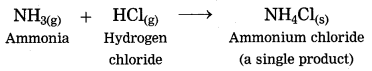(2) Magnesium burns in air to form white powder of magnesium oxide as a single product.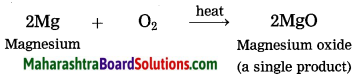(3) Iron reacts with sulphur to form iron sulphide.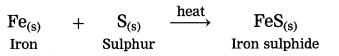Question 4.
What Is meant by a decomposition reaction?
The chemical reaction in which two or more products are formed from a single reactant is called decomposition reaction.

Question 5.
what is meant by a thermal decomposition?
The reaction in which a compound is decomposed by heating it to a high temperature is called thermal decomposition.

Question 6.
What is meant by a electrolytic decomposition?
The reaction in which a compound is decomposed by passing an electric current through its solution or molten mass is called an electrolytic decomposition.

Question 7.
Give two examples of thermal decomposition.
(1) At high temperature, calcium carbonate decomposes into calcium oxide and carbon dioxide.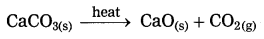(2) At high temperature sugar decomposes into black mass of carbon and water vapour.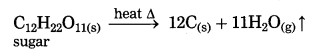Question 8.
Give an example of electrolytic decomposition.
When an electric current is passed through acidified water, it is electrolysed giving hydrogen
and oxygen.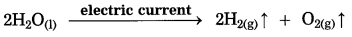Question 9.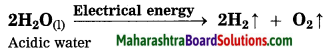(a) State the type of reaction.
(b) Define this reaction. (Practice Activity Sheet – 1)
(a) The type of reaction is electrolytic decomposition reaction.
(b) The reaction in which a compound is decomposed by passing an electric current through its solution or molten mass is called an electrolytic decomposition.

Question 10.
what is meant by a displacement reaction?
The reaction in which the place of the ion of a less reactive element in a compound is taken by another more reactive element by formation of its own ions, is called displacement reaction.

Question 11.
Give an example of displacement reaction.
when zinc granules are added to the blue coloured copper sulphate solution, the zinc ions formed from zinc atoms take the place or Cu2+ ions in CuSO4, and copper atoms, formed from Cu2+ ions comes out i.e. the more reactive zinc displaces the less reactive Cu from copper sulphate.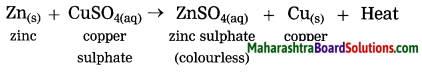Question 12.
Observe the reaction and answer the following questions.
CuSO4(aq) + Fe(s) → FeSO4(aq) + Cu(s)
(a) Identify and write the type of chemical reaction.
(b) write the definition of above reaction. (Practice Activity Sheet – 3)
(a) When iron powder is added to the blue coloured copper sulphate solution, the iron ions formed from iron atoms take the place or Cu2+ ions in CuSO4, and copper atoms, formed from Cu2+ ions comes out i.e. the more reactive iron displaces the less reactive Cu from copper sulphate. Therefore this reaction is a displacement reaction.

(b) The reaction in which the place of the ion of a less reactive element in a compound is taken by another more reactive element by formation of its own ions, is called displacement reaction.

Question 13.
what is meant by a double displacement reaction?
The reaction in which the ions in the reactants are exchanged to form a precipitate is called double displacement reaction.

Question 14.
Give two examples of double displacement reaction.
(1) Solutions of sodium chloride and silver nitrate react with each other forming a precipitate of silver chloride and a solution of sodium nitrate.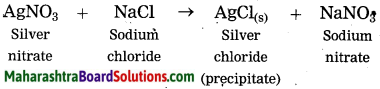White precipitate of AgCl is formed by exchange of ions Ag+ and Cl between the reactants.

(2) Barium suiphide reacts with zinc sulphate to form zinc sulphide and a white precipitate of barium sulphate.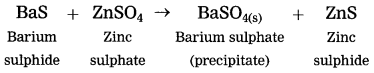white precipitate is formed by exchange of ions Ba++ and SO4 between the reactants.

Question 15.
Write down what you understand from the following chemical reaction:
AgNO3(aq) + NaCl(aq) → AgCl ↓ + NaNO3(aq)
(i) The above reaction is a double displacement reaction.
(ii) AgNO3 and NaCl are the reactants while AgCl and NaNO3 are the products.
(iii) The reactants and the product NaNO3 are in aqueous state. The product AgCl is formed in the form of precipitate.

Question 16.
Study the following chemical reaction and answer the questions given below:
AgNO3(aq) + NaCl(aq) → AgCl(s)↓ + NaNO3(aq)
(i) Identiry and write the type of chemical reaction.
(ii) write the definition of above type of chemical reaction.
(iii) Write the names of reactants and products of above reaction. (March 2019)
(i) The type of chemical reaction: Double displacement reaction.
(ii) The reaction in which the ions in the reactants are exchanged to form a precipitate is called double displacement reaction.
(iii)

1. The above reaction is a double displacement reaction.
2. AgNO3 and NaCl are the reactants while AgCl and NaNO3 are the products.
3. The reactants and the product NaNO3 are in aqueous state. The product AgCl is formed in the form of precipitate.

Question 17.
When sodium chromate solution is mixed with barium sulphate solution, a precipitate is formed.
(i) What is the colour of the precipitate formed?
(ii) Name the precipitate.
(iii) What is the type of chemical reaction?
(i) The colour of the precipitate is yellow.
(ii) The yellow precipitate formed is barium chromate.
(iii) The type of chemical reaction is double displacement.

Question 18.
Explain the term Exothermic reaction.
Exothermic reaction : The process in which heat is given out is called an exothermic reaction.
When NaOH(s) dissolves in water, there is evolution of heat leading to a rise in temperature.
NOH(s) + H3O(l) → NaOH(aq) + Heat

Question 19.
State whether the following reactions are exothermic or endothermic:
(i) 3CaO. Al2O3(s) + 6H2O(l) → 3CaO. Al2O3. 6H2O(s) + Heat
Exothermic reaction

(ii) 2CaSO4. H2O + 3H2O → 2CaSO4. 2H2O + Heat
Exothermic reaction

(iii) KNO3(aq) + H2O(l) + Heat → KNO3(aq)
Endothermic reaction

(iv) NaOH(s) + H2O(l) → NaOH(aq) + Heat
Exothermic reaction

(v) Transformation of ice into water.
Endothermic reaction

(vi) Water turns into ice.
Exothermic reaction

(vii) Cooking of food.
Endothermic reaction

(viii) Burning candle.
Exothermic reaction

Question 20.
What do you mean by slow speed reaction?
OR
Define: Slow speed reaction.
The reaction which requires long time for completion i.e. occurs slowly is called slow speed reaction.

Question 21.
What do you mean by fast speed reaction?
OR
Define: Fast speed reaction.
The reaction which is completed in short time i.e. occurs rapidly is called fast speed reaction.

Question 22.
Give two examples of slow speed reactions.
(1) On heating potassium chlorate (KClO3) it decomposes slowly into potassium chloride and oxygen gas.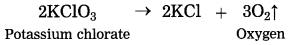This reaction requires long time for completion, therefore it is slow speed reaction.

(2) Rusting of iron is a slow speed reaction. In this reaction iron reacts with oxygen from air to form iron oxide.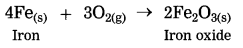Question 23.
Give two examples of fast speed reactions.
(1) The reaction between sodium hydroxide (NaOH) and hydrochloric acid (HCl) is a neutralization reaction and it is fast speed reaction.
NaOH2(aq) + HCl(aq) → NaCl(aq) + H2O(l)
This neutralizaton reaction is completed in short time, therefore it is fast speed reaction.

(2) Aqueous solution of sodium chloride reacts with silver nitrate solution to form white precipitate of silver chloride (NaCl) and sodium nitrate.
NaCl(aq) + AgNO3(aq) → NaNO3(aq) + AgCl ↓
This reaction is completed in short time, therefore it is fast reaction.

Question 24.
Write a short note on slow speed and fast speed reactions.
Slow speed reaction:
The reaction which requires long time for completion i.e. occurs slowly is called slow speed reaction.
Examples:
(1) On heating potassium chlorate (KClO3) it decomposes slowly into potassium chloride and oxygen gas.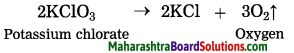This reaction requires long time for completion, therefore it is slow speed reaction.

(2) Rusting of iron is a slow speed reaction. In this reaction iron reacts with oxygen from air to form iron oxide.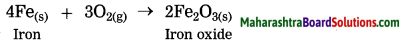This reaction requires long time for completion.

Fast speed reaction:
The reaction which is completed in short time i.e., occurs rapidly is called fast speed reaction.
Examples:
(1) The reaction between sodium hydroxide (NaOH) and hydrochloric acid (HCl) is a neutralization reaction and it is fast speed reaction.
NaOH(aq) + HCl(aq) → NaCl(aq) + H2O(l)
This neutralizaton reaction is completed in short time, therefore it is fast speed reaction.

(2) Aqueous solution of sodium chloride reacts with silver nitrate solution to form white precipitate of silver chloride (NaCl) and sodium nitrate.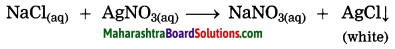This reaction is completed in short time, therefore it is fast reaction.

Question 25.
State the factors which affect the speed (or rate) of a reaction.
The factors which affect the rate of a reaction are

1. Nature of the reactants.
2. Size of the particles of the reactants.
3. Concentration of the reactants.
4. Temperature of the reaction.
5. Catalyst.

Question 26.
How does the rate of reaction depend on the nature of the reactants? Illustrate with suitable example.
(1) when the reactant combines with two or more other reactants then the rate of a chemical reaction depends on the nature of the reactants.

(2) Both Al and Zn reacts with dilute hydrochloric acid, H2 gas is liberated and water soluble salts of these metals are formed. However, aluminium metal reacts faster with dil. HCl as compared to zinc metal.

(3) Al is more reactive than Zn. Therefore, the rate of reaction of Al with hydrochloric acid is higher than that of Zn. Hence, the nature of the reactant affect the rate of a reaction.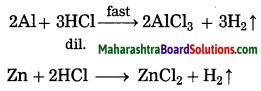Question 27.
How does the rate of a reaction depend on the size of the particles of reactants?
(1) In the reaction of dil. HCl and Shahabad tile, CO2 effervescence is formed slowly. On the other hand, C2 effervescence forms at faster speed with the powder of Shahabad tile.

(2) The above observation indicates that the rate of a reaction depends upon the size of the particles of the reactants taking part in the reaction. Smaller the size of the reactant particles taking part in a reaction faster will be the rate of reaètion.

Question 28.
How does the rate of a reaction depend upon the concentration of the reactants? Give suitable example.
(1) A chemical reaction takes place due to collisions of the reactant molecules. Higher the concentrations of the reactants more will be the frequency of collisions and faster wifi be the rate of the reaction.

(2) In the reaction of dil. HCl and CaCO3, CaCO3 disappears slowly and CO2 also liberates slowly. On the other hand the reaction with concentrated HCl takes place rapidly and CaCO3 disappears fast.

(3) Concentrated acid reacts faster than dilute acid, that means the rate of a reaction is proportional to the concentration of reactants.

Slow reaction:
CaCO3 + dli.2HCl → CaCl2 + CO2 + H2O
Fast reaction: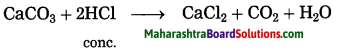Question 29.
How does the rate of a reaction depend upon the temperature of reactants? Give suitable example.
(i) (1) When the temperature of the reactants is increased, the reactant molecules start moving with more velocity and their kinetic energy increases. As a result, the number collisions increases. Hence, the rate of chemical reaction increases.

(2) Lime stone on heating decomposes to give CO2, which turns 1ime water milky. On the other hand, the lime water does not turn milky before heating the lime stone: because of the zero rate of reaction. The above observation indicates that the rate of a reaction increases on increasing the temperature.

(ii) Solid CaCO3 does not decompose at room temperature when heated, it decomposes to give CaO and CO2 that means the rise in temperature increases the rate of reaction. CaCO3 room temperature No chemical reaction.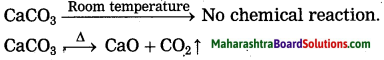Question 30.
How does the rate if a reaction depend upon the catalyst? Give suitable example.
(1) The substance in whose presence the rate of a chemical reaction changes, without causing any chemical change to it is called a catalyst.

(2) On heating potassium chlorate (KClO3) decomposes into potassium chloride and oxygen slowly.
2KClO3Δ2KCl+3O2
The rate of the above reaction neither increases by reducing the particle size nor by increasing the reaction temperature. However in the presence of manganese dioxide, KClO3 decomposes at a comparatively lower temperature and oxygen is produced more briskly. No chemical change takes place in MnO2 in this reaction. It acts as catalyst.

Question 31.
State the Importance of rate in a chemical reaction.

1. The use of strong acid and strong base in a chemical reaction increases the rate of reaction.
2. In a chemical reaction, if the smaller size of the reactant particles, the concentrated solution, high temperature and use of catalyst increases the rate of chemical reaction.
3. The rate of chemical reaction is important with respect to environment.
4. If the rate of chemimal reaction is fast it is profitable for the chemical factories.
5. The ozone layer in the earth’s atmosphere protects the life of earth from the ultraviolet radiation of the sun. The process of depletion or maintenance of this layer depends upon the rate of production or destruction of ozone molecules.

Question 32.
Define Oxidation reaction.
Oxidation: The chemical reaction in which a reactant combines with oxygen or loses hydrogen to form the product is called oxidation reaction.

Question 33.
Give examples of oxidation.
(1) when carbon burns in air, it forms carbon dioxide. In this reaction carbon accepts oxygen, therefore, this is an oxidation reaction.
C(s) + O2(g) → CO2(g)

(2) when sodium reacts with ethyl alcohol, sodium ethoxide and hydrogen gas is formed. In this reaction, hydrogen is removed from ethyl alcohol, therefore this is an oxidation reaction.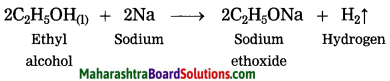(3) Acidified potassium dichromate: (K2Cr2O7/H2SO4) oxidises ethly alcohol to acetic acid.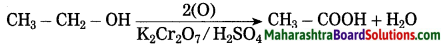Question 34.
What do you mean by oxidant? Explain with suitable example.
The chemical substances which bring about an oxidation reaction by making oxygen available are called oxidants or oxidizing agents.

1. In the combustion of carbon, oxygen is an oxidant.
2. In the oxidation of ethly alcohol, potassium dichromate is used as oxidant.

Question 35.
Name the various oxidants. How nascent oxygen is liberated from these oxidants?
K2Cr2O7/H2SO4, KMnO4/H2SO4 are the commonly used chemical oxidants. Hydrogen peroxide (H2O2) is used as a mild oxidant. Ozone (O3) is also a chemical oxidant. Nascent oxygen is generated by chemical oxidants and it is used for the oxidation reaction.
O3 → O2 + [O].
H2O2 → H2O + [O]
K2Cr2O7 + 4H2SO4 → K2SO4 + Cr2(SO4)3 + 4H2O + 3[O]
2KMnO4 + 3H2SO4 → K2SO4 + 2MnSO4 + 3H2O + 5[O]
Nascent oxygen is a state prior to the formation of the O2 molecule. It is the reactive form of oxygen and is represented by the symbol as [O]

Question 36.
Acidified potassium permanganate (KMnO4) is a chemical oxidant and explain, how acidified potassium permanganate oxidise ferrous sulphate (FeSO4). Accordingly write a new definition of oxidation and reduction.
Acidified KMnO4 oxidises ferrous sulphate (FeSO4) to ferric sulphate Fe2(SO4)3 and in addition to above K2SO4 and MnSO4 by-products are formed.
2KMnO4 + 10 FeSO4 → 8H2SO4 → K2SO4 + 2MnSO4 + 5Fe2(SO4)3 + 8H2O
2FeSO4 → Fe2(SO4)3
Ionic reaction :
2Fe2 + 2SO42+ → 2Fe3+ + 3SO22-
Net Ionic equation Fe2+ → Fe3+ + e
When ferric ion is formed from ferrous ion the positive change is increased by one unit. While this happens the rerrous ion loses one electron.

When metal or its ion loses electron, it is called an oxidation and gain of electron is called reduction.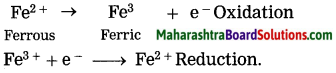Question 37.
Define reduction reaction.
The chemical reaction in which a reactant gains hydrogen and loses oxygen to form the product is called the reduction reaction.

Question 38.
Give two examples of reduction.
(1) When hydrogen gas is passed over black copper oxide a reddish coloured layer of copper is formed.
In this reaction an oxygen atom removed from CuO to form copper, hence, this is reduction.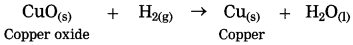(2) When hydrogen gas is passed over red hot coke, methane is obtained.
Here, hydrogen is added to coke (carbon). Hence, this is reduction.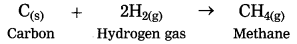Question 39.
What do you mean by reductant? Explain with suitable example.
The chemical substances which bring about reduction by making hydrogen available are called reductant. In the preparation of methane from carbon, hydrogen is a reductant.

Question 40.
What are redox reactions? Identify the substances that are oxidised and the substances that are reduced in the following reactions:
(1) 2H2S2(g) + SO2(g) → 3S(s) + 2H2O(l)
(2) CuO(s) + H2(g) → CU(s) + H2O(l)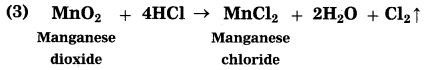When oxidation and reduction take place simultaneously in a given chemical reaction, it is known as a redox reaction.
(1)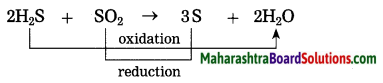H2S is oxidised and SO2 is reduced.

(2)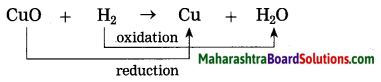CuO is reduced and H2 is oxidised.

(3)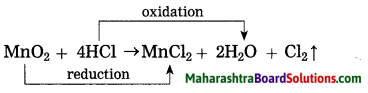(1) Oxidation: H2S, H2O and HCl.
(2) Reduction: SO2, CuO and MnO2

Question 41.
Observe the following reaction and answer the questions given below:
BaSO4 + 4C → BaS + 4CO
(1) what type of reaction is it? Justify.
(2) Give one more example.
(1) This is a redox reaction. In this reaction the reduction of BaSO4 and oxidation of carbon take place simultaneously.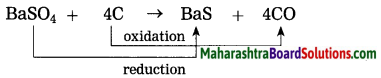(2) Example
CuO+H2 → Cu + H2O
2H2S + SO2 → 3S + 2H2O

Question 42.
What is corrosion?
The slow process of decay or oxidation of metals due to various components of atmosphere is known as corrosion.
Iron rusts and a reddish coloured layer is collected on it. This is corrosion of iron.

Question 43.
How does rusting of iron occur?
Iron when exposed to moist air forms a reddish layer of hydrated ferric oxide.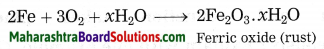Question 44.
How can corrosion be prevented?

1. Corrosion damages buildings, bridges, automobiles, ships, iron railings and other articles made of iron.
2. It can be prevented by using an anti-rust solution, coating the surface by a paint, processes like galvanising and electroplating with other metals.

Question 45.
What is corrosion? Do gold ornaments corrode? Justify.
The slow process of decay or oxidation or metal due to the effect of air, moisture and acids on
it is known as corrosion.
(1) Gold is a noble metal. There is no effect or moist air or action of acid on it at any temperature.
(2) Pure gold is a very soft metal. it breaks and gets bent easily. Hence, in gold ornaments, gold is alloyed with other metals 1ike copper or silver in appropriate proportion to make it hard and resistant to corrosion. Hence gold ornaments do not get corroded.

Question 46.
Complete the process of iron rusting by filling the blanks. Suggest a way to prohibit the process.
The iron rust is formed due to reaction. Different regions on iron surface become anode and cathode.
Reaction on anode region:
Fe(s) → Fe2+ (aq) + 2e
Reaction on cathode region:
O2(g) + 4H+ (aq) +………→ 2H2O(l)
when Fe2+ ions migrate from anode region they react with……..to fomm Fe3+ ions.
A reddish coloured hytirated oxide is formed from……….ions. It is called rust.
2Fe3+ (aq) + 4H2O(l) → +………+ 6H+ (aq)
A way to prevent rusting………..
(Practice Activity Sheer – 2)
The iron rust is formed due to electrochemical reaction. Different regions on iron surface become unode and cathode.
Reaction on anode region:
Fe(s) → Fe2+ (aq) + 2e
Reaction on cathode region:
O2(g) + 4H+ (aq) + 4e → 2H2O(l)
when Fe2+ ions migrate from anode region they react with water to form Fe3+ ions.
A reddish coloured hydrated oxide is formed from Fe3+ ions. It is called rust.
2Fe3+ (aq) + 4H2O(l) → + Fe2O3. H2O(s) + 6H+(aq)
A way to prevent rusting by colouring with acrylic paints, Zn plating, galvanizing, anodizing, alloying, etc.

Question 17.
Deifne: Rancidity.
When oil or fat or left over cooking oil for making food stuff undergoes oxidation ir stored for a long time and it is found to have foul odour called rancidity.

Distinguish between the following:

Question 1.
Combination reaction and Decomposition reaction.

 Combination reaction Decomposition reaction 1. In a combination re­action, two or more reactants take part in the chemical reaction. 1. In a decomposition reaction there is only one reactant in the chemical reaction. 1. In the combination reaction, only one product is formed. 2. In a decomposition reaction, two or more products are formed.

Question 2.
Oxidation and reduction

 Oxidation Reduction 1. The chemical reaction in which reactants gain oxygen or lose hydrogen is called oxidation. 1. The chemical reaction in which reactants gain hydrogen or lose oxygen is called reduction. 2. A reducing agent undergoes oxidation. 2. An oxidising agent undergoes reduction.

Question 3.
Exothermic and Endothermic reaction.

 Exothermic reaction Endothermic reaction 1. The reaction in which heat is evolved is called an exothermic reaction. 1. The reaction in which heat is absorbed is called an endothermic reaction. 2. The evolution of heat leads to a rise in the temperature of the solution. 2. The absorption of heat leads to a fall in the temperature of the solution.

Give scientific reasons:

Question 1.
Grills of doors and windows are always painted before they are used.

• Grills of doors and windows are made from iron. Iron has a tendency to undergo corrosion.
• Paint does not allow air or moisture to come in contact with iron surface.
Therefore, to prevent rusting of iron. grills of doors and windows are always painted before they are used.

Question 2.
Physical states of reactants and products are mentioned while writing a chemical equation.
(1) while writing a chemical equation, gaseous, 1iquid and solid states are symbolised as (g), (l) and (s) respectively.

(2) This is done to make it more informative and to emphasise that those reactions occur in that manner only under those conditions. Hence, physical states of reactants and products are mentioned while writing a chemical equation.

Question 3.
Iron articles rust readily whereas steel which is also mainly made of iron does not undergo corrosion.
(1) Iron articles rust readily as iron reacts with oxygen and moisture of air to convert into its hydroxide and oxide (Fe2O3. x H2O), while steel is an alloy of iron, carbon and chromium.

(2) The properties of an alloy are different from the properties of its constituents. The added metals increase its resistance to corrosion. It is more durable and clean.

Question 4.
Concentrated hydrochloric acid reacts more vigorously with calcium carbonate than dilute hydrochloric acid.

1. The rate of a reaction increases with the concentration of the reactant.
2. As concentrated hydrochloric acid contains more number of HCl molecules than those in an equal volume of dilute HCl, concentrated HCl reacts more vigorously with calcium carbonate.

Question 5.
Zinc powder reacts much faster with dil. H2SO4 than does granulated zinc of the Same mass.
(1) In a reaction. the rate of the reaction depends upon the particle size of the solid reactant as the reaction takes place on the surface only. Smaller the particles are, the more will be their total surface area and faster will be the rate of the reaction.
(2) Hence, zinc powder reacts much faster with dil. H2SO4 than does granulated zinc.

Question 6.
When copper articles exposed to air for a long time, gets corroded.
Copper oxidises to form black coloured laver of copper oxide. when copper oxide combines with carbon dioxide from air, copper loses its lustre due to formation of greenish layer of copper carbonate on its surface. Thus, copper articles exposed to air for a long time get corroded.

Question 7.
When silver vessels exposed to air turns blackish after sometime.
On exposure to air, silver vessels turns blackish after sometime. This is because of the layer of silver sulphide (Ag2S) formed by the reaction or silver with hydrogen suphide in air.

Explain the following reactions giving their balanced chemical equations:

Question 1.
Calcium carbonate (Lime stone) is heated.
When calcium carbonate (Lime stone) is heated at high temperature it decomposes to form quicklime and carbon dioxide gas.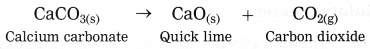Question 2.
Copper reacts with dil. nitric acid.
When copper reacts with dil. nitric acid, nitric oxide gas is formed.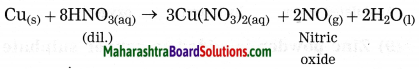Question 3.
Copper reacts with conc. nitric acid.
When copper reacts with conc. nitric acid, reddish coloured poisonous nitrogen dioxide gas is formed.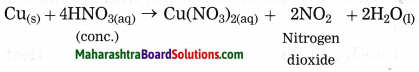Question 4.
Ammonia gas reacts with hydrogen chloride gas.
When ammonia gas reacts with hydrogen chloride gas, it forms the salt ammonium chloride in gaseous state, but immediately it got transformed into the solid state.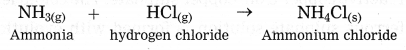Question 5.
Magnesium strip is burnt in air.
When magnesium strip is burnt in air, a white powder of magnesium oxide is formed.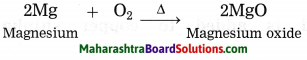Question 6.
Calcium oxide is mixed with water.
When calcium oxide (slaked lime) is mixed with water, calcium hydroxide is formed with evolution of large amount of heat.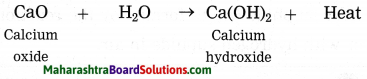Question 7.
Sugar is heated.
When sugar is heated, it decomposes to form carbon (black substance).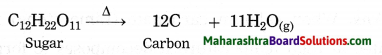Question 8.
Electric current is passed through acidulated water.
When an electric current is passed through acidulated water, it decomposes into hydrogen and
oxygen gas.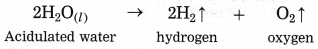Question 9.
Zinc powder is added to copper sulphate solution.
When zinc powder is added to copper sulphate solution, more reactive zinc displaces less reactive copper from copper sulphate solution. The colourless zinc sulphate is formed with evolution of heat.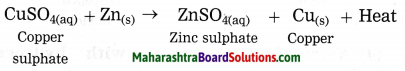Question 10.
Iron powder is added to copper sulphate solution.
When iron powder is added to copper sulphate solution, more reactive iron displaces less reactive copper from copper sulphate. The colourless ferrous sulphate solution is formed with evolution of heat.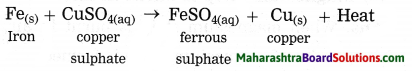Question 11.
When lead is added to copper sulphate solution, more reactive lead displaces less reactive copper from copper sulphate. The colourless lead sulphate solution is formed with evolution of heat.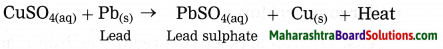Question 12.
Potassium chromate solution is added to barium sulphate solution.
When potassium chromate solution is added to barium sulphate solution, yellow precipitate of barium chromate is formed.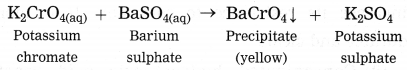Question 13.
Calcium chloride solution is added to sodium carbonate solution.
When calcium chloride solution is added to sodium carbonate solution, white precipitate of calcium carbonate is formed.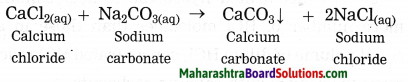Question 14.
Sodium chloride solution is mixed with silver nitrate solution.
When sodium chloride solution is mixed with silver nitrate solution, white precipitate or silver chloride is formed.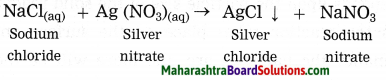Question 15.
Dilute sulphuric acid is added to barium chloride solution.
When dilute sulphuric acid is added to barium chloride solution, white precipitate of barium sulphate is rormed.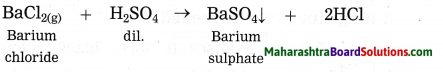Question 16.
Calcium carbonate (Lime stone) is treated with dil. hydrochloric acid.
When calcium carbonate (lime stone) is treated with dil. hydrochloric acid, carbon dioxide gas is formed.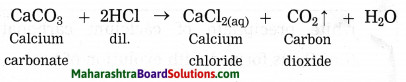Question 17.
Aluminium is treated with dil. hydrochloric acid.
When aluminium is treated with dilute hydrochloric acid, hydrogen gas is liberated.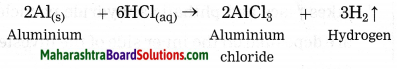Question 18.
Magnesium is treated with hydrochloric acid.
When magnesium is treated with hydrochloric acid, hydrogen gas is liberated.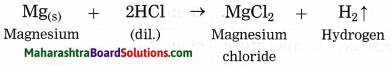Question 19.
Hydrogen peroxide is decomposed in the presence of manganese dioxide (MnO2).
When hvdrogen peroxide is decomposed in the presence of manganese dioxide (MnO2), water and oxygen are formed.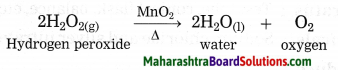Question 20.
Ethyl alcohol is treated with acidified potassium dlchromate.
When ethly alcohol is treated with acidified potassium dichromate, acetic acid is formed. This is oxidation reaction.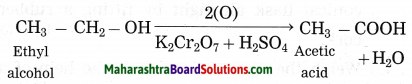Question 21.
Hydrogen gas is passed over black copper oxide.
When hydrogen gas is passed over black copper oxide, a reddish coloured layer of copper is formed.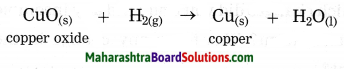## निष्कर्ष

आम्हाला आशा आहे की महाराष्ट्रावरील हा लेख तुम्हाला 'दहावी विज्ञान धडा रासायनिक अभिक्रिया आणि समीकरणे स्वाध्याय' मदत करेल. तुम्हाला काही प्रश्न असल्यास, त्यांना खाली टिप्पणी विभागात मोकळ्या मनाने पोस्ट करा. आम्ही लवकरात लवकर तुमच्याकडे परत येऊ.
Share: